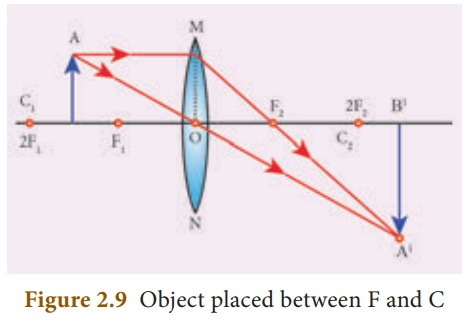Home | | Science 10th Std | Refraction Through a Convex Lens

# Refraction Through a Convex Lens

Let us discuss the formation of images by a convex lens when the object is placed at various positions.

REFRACTION THROUGH A CONVEX LENS

Let us discuss the formation of images by a convex lens when the object is placed at various positions.

### Object at infinity

When an object is placed at infinity, a real image is formed at the principal focus. The size of the image is much smaller than that of the object (Figure 2.6).### Object placed beyond C (>2F)

When an object is placed behind the center of curvature(beyond C), a real and inverted image is formed between the center of curvature and the principal focus. The size of the image is the same as that of the object (Figure 2.7).### Object placed at C

When an object is placed at the center of curvature, a real and inverted image is formed at the other center of curvature. The size of the image is the same as that of the object (Figure 2.8).### Object placed between F and C

When an object is placed in between the center of curvature and principal focus, a real and inverted image is formed behind the center of curvature. The size of the image is bigger than that of the object (Figure 2.9).### Object placed at the principal focus F

When an object is placed at the focus, a real image is formed at infinity. The size of the image is much larger than that of the object (Figure 2.10).### Object placed between the principal focus F and optical centre OWhen an object is placed in between principal focus and optical centre, a virtual image is formed. The size of the image is larger than that of the object (Figure 2.11).Study Material, Lecturing Notes, Assignment, Reference, Wiki description explanation, brief detail
10th Science : Chapter 2 : Optics : Refraction Through a Convex Lens |